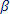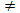## Summary

Keep in mind, there is a difference between the strength of the relationship and the strength of evidence against the null hypothesis...

### Technology Skills learned in this lab

• Generating a scatterplot
• Calculating the correlation coefficient
• Determining the least squares regression line
• Obtaining the test statistic and p-value for testing Ho:= 0 vs. Ha:0
• Approximating a 95% confidence interval for a population slope

### Statistics Skills learned in this lab

• Describing a scatterplot in terms of direction, strength, and form
• Evaluating a correlation coefficient
• Interpretating the coefficients (intercept and slope) in a regression equation
• Interpreting the standard error of a regression slope
• Interpreting and evaluating a p-value in the regression setting
• Interpreting a confidence interval for a population slope